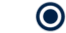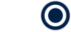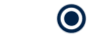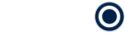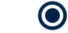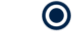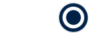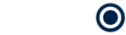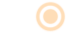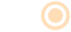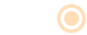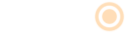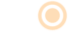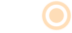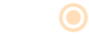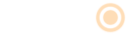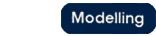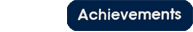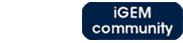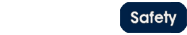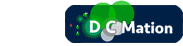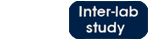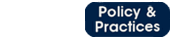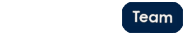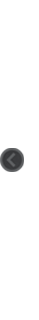# Team:Oxford/calculating parametersParameter Calibration

# Parameter calibration and data fitting

Characterization of a biological system is an extremely important area of engineering in synthetic biology. Part of this characterization involves deducing the structure of the system, while another component of this involves matching experimental data to theoretical data and calculating the defining parameters from this. To do this accurately and quickly, we have developed a script which calculates the value of the given variable that gives the closest fit to the experimental data.

The programme requires a theoretical input form with one variable parameter to be compared with experimental data. After this, simulations are run for a range of parameter values and the programme finds the parameter value which maximizes the closeness of fit. This is done through the use of the Euclidean Norm of the vector calculated from the difference between the experimental data points and the hypothesized relationship. The Euclidean Norm is defined as: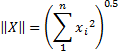• n = number of data points available
• x_i= difference between theoretical form and data point for i^th data point.

• This code was very useful in a number of situations, for example in characterizing the bead diffusion parameters from given data. Displayed below is experimental diffusion data plotted alongside our fitted theoretical form.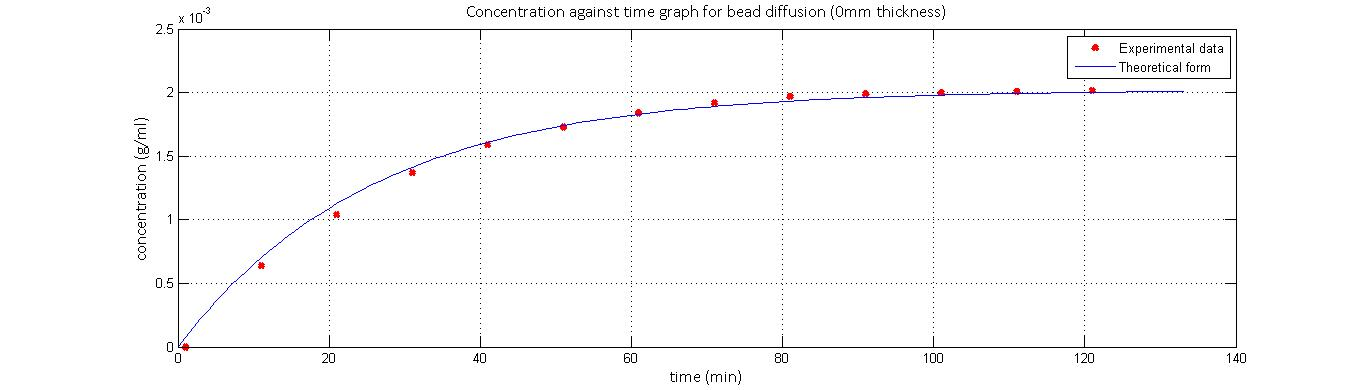Oxford iGEM 2014